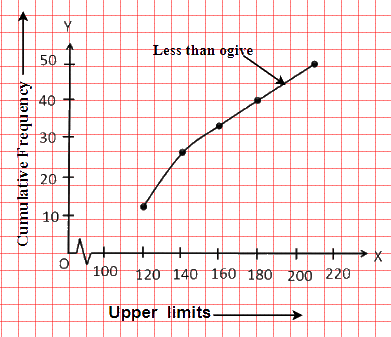# Ex.14.4 Q1 STATISTICS Solution - NCERT Maths Class 10

Go back to  'Ex.14.4'

## Question

The following distribution gives the daily income of  $$50$$ workers of a factory.

 Daily income (in Rs) $$100 - 120$$ $$120 - 140$$ $$140 - 160$$ $$160 – 180$$ $$180 - 200$$ Number of workers $$12$$ $$14$$ $$8$$ $$6$$ $$10$$

Convert the distribution above to a less than type cumulative frequency distribution, and draw its ogive.

## Text Solution

What is known?

The daily income of $$50$$ workers of a factory.

What is unknown?

The less than type cumulative frequency distribution and its ogive.

Reasoning:

The representation of cumulative frequency distribution graphically is known as a cumulative frequency curve, or an ogive.

Steps:

The frequency distribution table of less than type is as follows:

 Daily income (in Rs) (Upper class Limits) Cumulative Frequency Less than $$120$$ $$12$$ Less than $$140$$ $$12 + 14 = 26$$ Less than $$160$$ $$26 + 8 = 34$$ Less than $$180$$ $$34 + 6 = 40$$ Less than $$200$$ $$40 + 10 = 50$$

Taking upper class limits of class intervals on $$x$$-axis and their respective frequencies on $$y$$-axis, its ogive can be drawn as follows:Learn from the best math teachers and top your exams

• Live one on one classroom and doubt clearing
• Practice worksheets in and after class for conceptual clarity
• Personalized curriculum to keep up with school